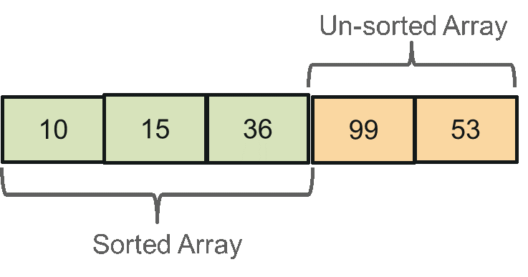# How To Implement Selection Sort in Java?Selection sort is one of the simplest algorithms to learn & code. This article will help you to get into the details of Selection Sort In Java. Following pointers will be covered in this article,

So let us get started with this Selection Sort In Java article,

The most important part in Selection sort is to understand that the algorithm maintains two sub-arrays:

• One sub-array is the sorted array
• Another sub-array is the unsorted arraySorted sub-array is kept at the start of the original array whereas the rest of the part forms the un-sorted sub-array. The algorithm moves the smallest element from the unsorted array at the end sorted array.
To be precise, this is not moving, it is swapping of the smallest elements of the un-sorted array with the first element of the un-sorted array, and then increasing the index of the sorted array.

Let’s make it simpler. Selection sort first finds the smallest element in the unsorted array (array[0..n], which is the complete array in first iteration) and swaps it with the first element. Then it finds the second smallest element in the unsorted array (i.e. array[1..n]) and swaps it with the second element, and the algorithm keeps doing this until the entire array is sorted.

So, the sorted array grows from 0 to n with each iteration and the un-sorted array reduces form n to 0 with each iteration. As the algorithm continuously selects the smallest elements & swaps it into its correct position, thus it is named as Selection Sort.
As time complexity is one of the most important factors in analysing the efficiency of the algorithm, let’s look at the time complexity of Selection Sort.

• Worst Case Complexity: O(n2)
• Best Case Complexity: O(n2)
• Average Case Complexity: O(n2)

Moving on with this article on Selection Sort in Java

Selection Sort Algorithm

Step 1 − Set Min_Index to 0
Step 2 − Search for the smallest element in the array
Step 3 − Swap with value with the element at the Min_Index
Step 4 − Increment Min_Index to point to next element
Step 5 − Repeat until the complete array is sorted

Moving on with this article on Selection Sort in Java

## Selection Sort Example

xarray[] = 15 10 99 53 36

Find the smallest element in array[0…4] & swap it with the element at beginning
10 15 99 53 36

Find the smallest element in arr[1…4]. As 15 is the next smallest element, move to next element.
10 15 99 53 36

Find the minimum element in arr[2…4] & & swap it with the element third element
10 15 36 53 99

Find the smallest element in arr[1…4]. As 53 is the next smallest element, move to next element.
10 15 36 53 99

The last element is by default at its correct position.
10 15 36 53 99

Now that we understand the working of Selection Sort algorithm, let’s understand how to implement Selection Sort in Java.

## Selection Sort Method in Java

```void sort(int array[])
{
int n = array.length;
// Loop to increase the boundary of the sorted array
for (int i = 0; i < n-1; i++)
{
// Finding the smallest element in the unsorted array
int min_element = i;
for (int j = i+1; j < n; j++)
if (array[j] < array[min_element])
min_element = j;
/* Swap the smallest element from the unsorted array with the last element of the sorted array */
int temp = array[min_element];
array[min_element] = array[i];
array[i] = temp;
}
}
```

Finally let’s look at the complete Java program to perform Selection Sort.

## Selection Sort Program in Java

```class SelectionSort
{
// Selection Sort Method
void sort(int array[])
{
int n = array.length;
for (int i = 0; i < n-1; i++)
{
int min_element = i;
for (int j = i+1; j < n; j++)
if (array[j] < array[min_element])
min_element = j;
int temp = array[min_element];
array[min_element] = array[i];
array[i] = temp;
}
}
// Method to print the elements of an array
void printarrayay(int array[])
{
int n = array.length;
for (int i=0; i<n; ++i)
System.out.print(array[i]+" ");
System.out.println();
}
// Main Method
public static void main(String args[])
{
SelectionSort ob = new SelectionSort();
int array[] = {15, 10, 99, 53, 36};
ob.sort(array);
System.out.println("Sorted arrayay");
ob.printarrayay(array);
}
}
```

Output:Now after executing the above Java program you would have understood how Selection Sort works & how to implement it in Java. I hope this blog is informative and added value to you. Thus we have come to an end of this article on ‘Selection Sort in Java’. If you wish to learn more, check out the Java Course Training by Edureka, a trusted online learning company. Edureka’s Java J2EE and SOA training and certification course is designed to train you for both core and advanced Java concepts along with various Java frameworks like Hibernate & Spring.

Got a question for us? Please mention it in the comments section of this blog  and we will get back to you as soon as possible.

Upcoming Batches For Java Certification Training Course
Course NameDate
Java Certification Training Course

Class Starts on 29th October,2022

29th October

SAT&SUN (Weekend Batch)
View Details
Java Certification Training Course

Class Starts on 26th November,2022

26th November

SAT&SUN (Weekend Batch)
View DetailsREGISTER FOR FREE WEBINARThank you for registering Join Edureka Meetup community for 100+ Free Webinars each month

### Subscribe to our Newsletter, and get personalized recommendations.#### How To Implement Selection Sort in Java?

edureka.co